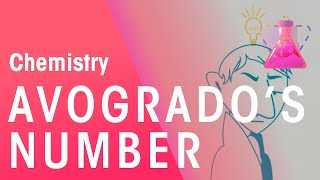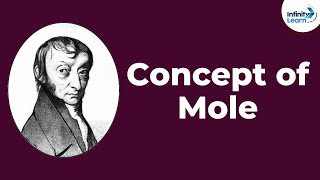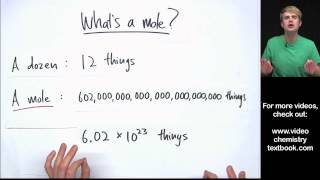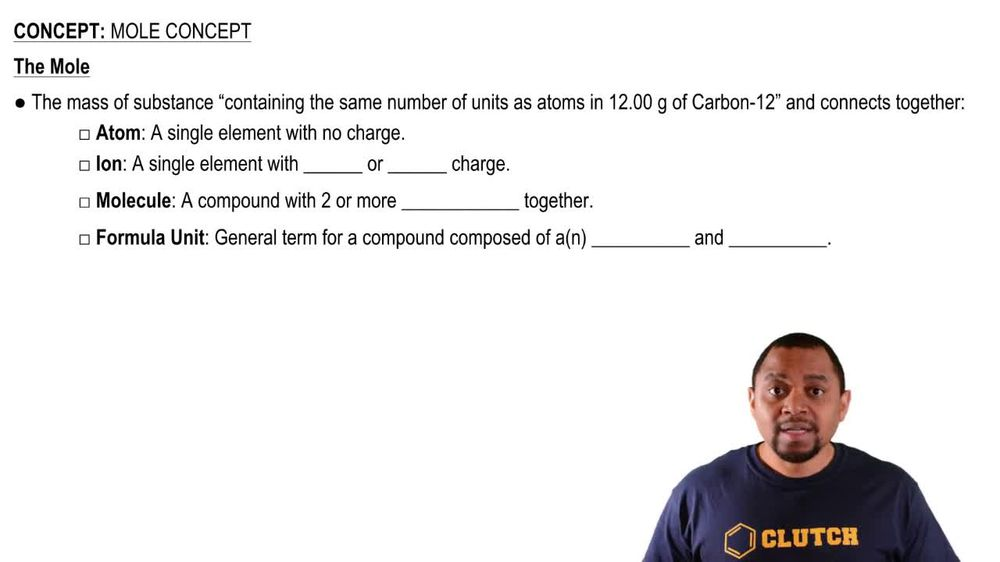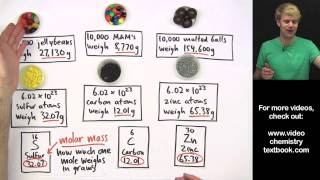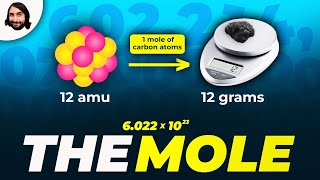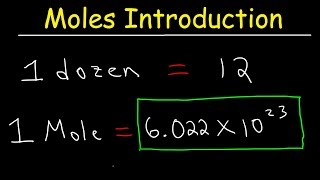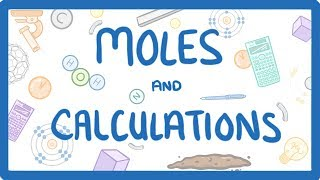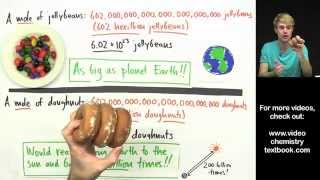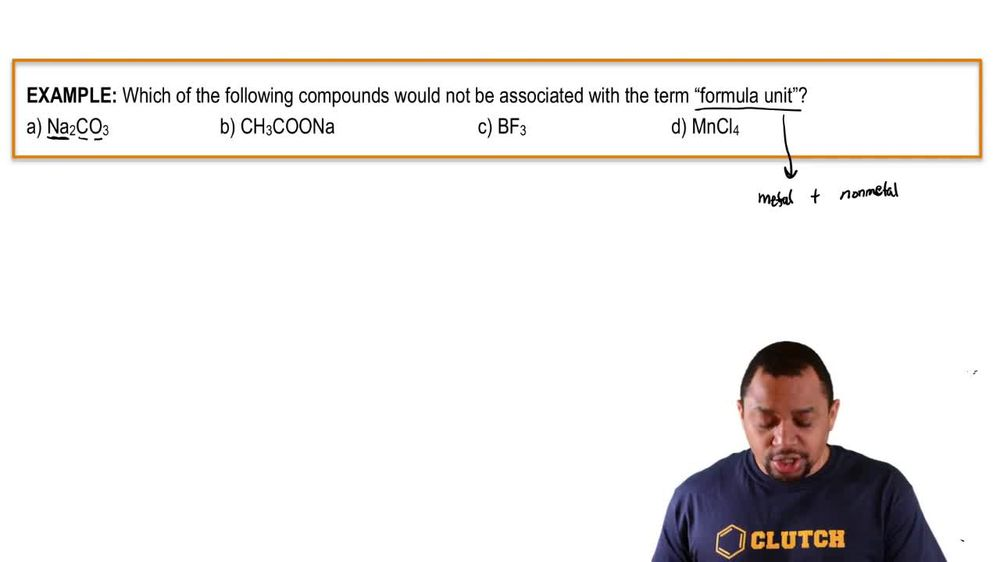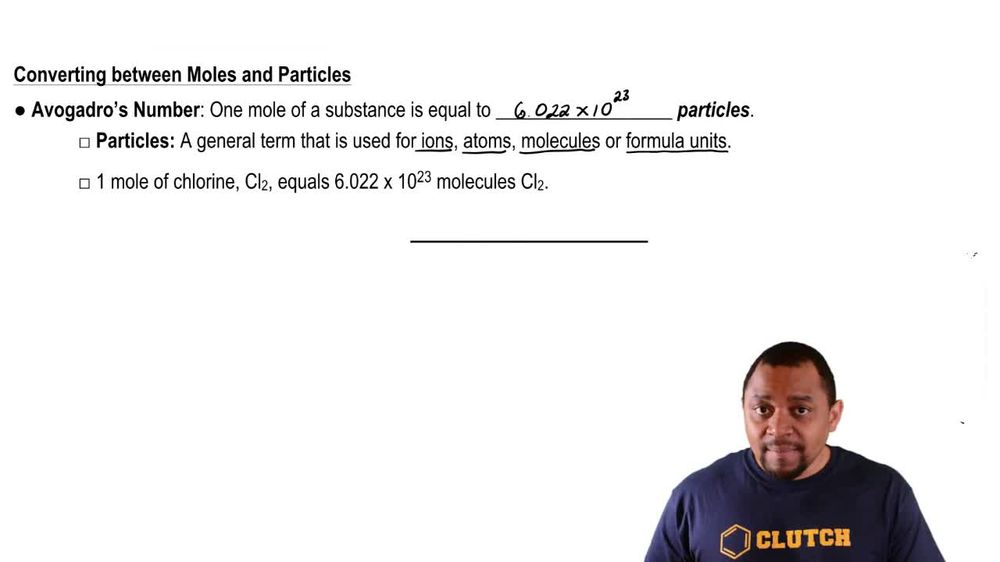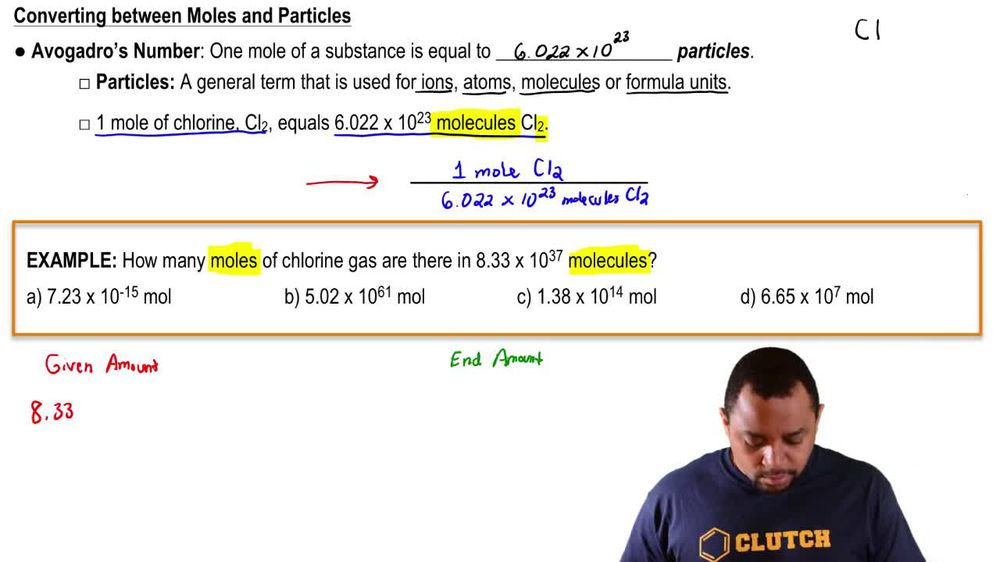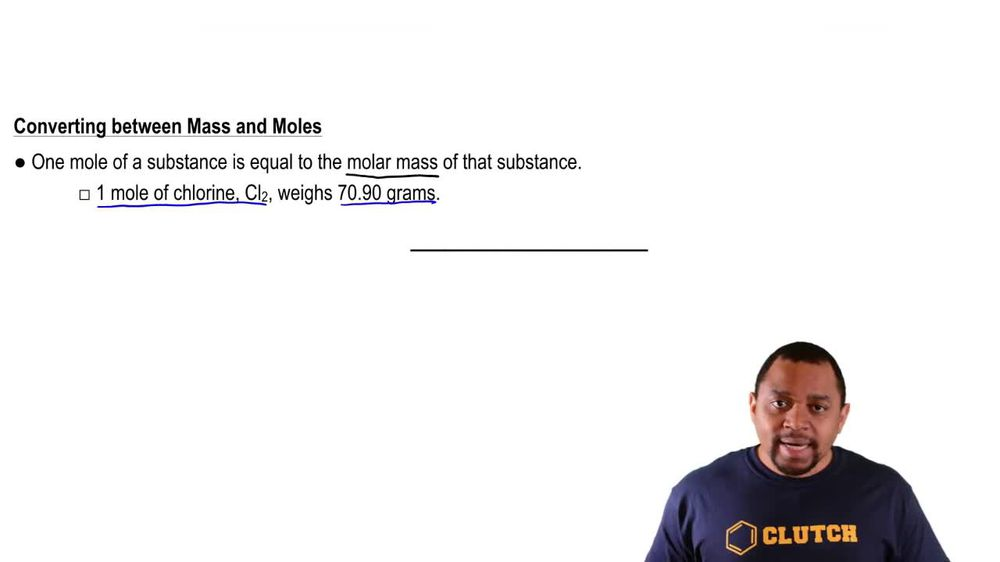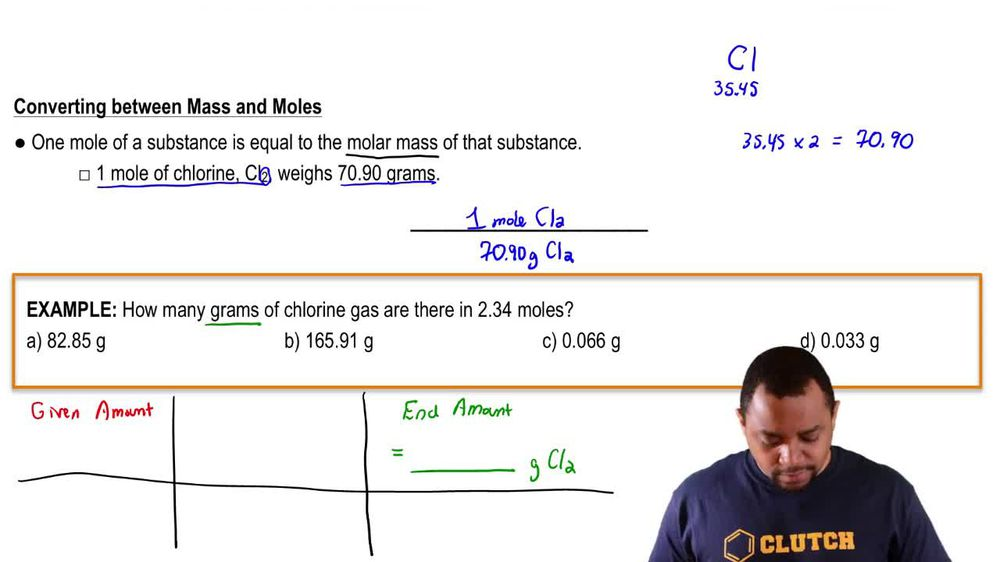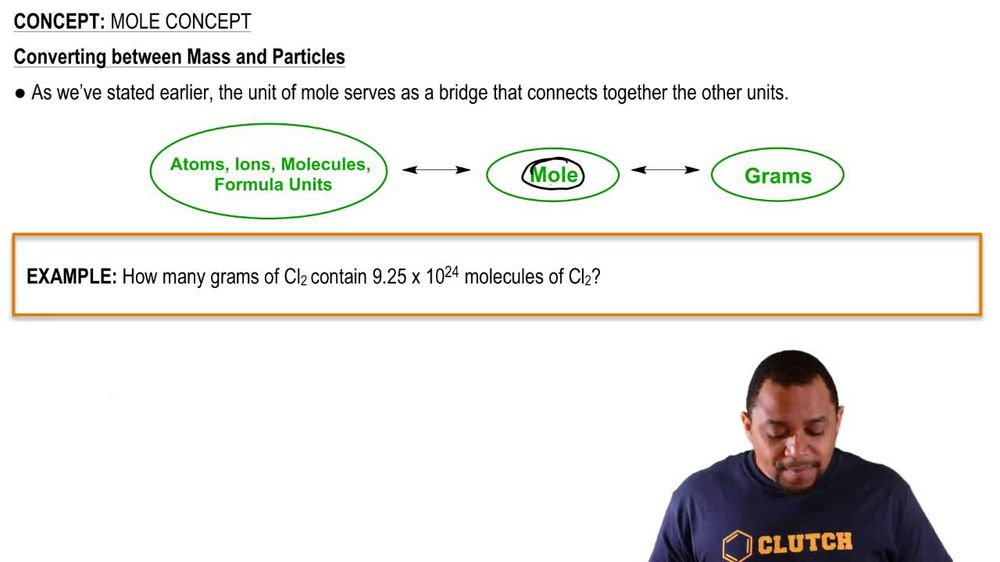Start typing, then use the up and down arrows to select an option from the list.
1. 2. Atoms & Elements2. Mole Concept
Problem

# How many molecules (or formula units) are in each sample? a. 85.26 g CCl4 b. 55.93 kg NaHCO3 c. 119.78 g C4H10 d. 4.59 * 105 g Na3PO4

Relevant Solution4m
Play a video:
Hey everyone welcome back. So let's get started with this video. So here we're told consider 100 and 2.3 mg sample of a compound with the molecular formula of C 10 H 12 04. And they wanted to calculate the malls and the molecules of the compound in the sample. Okay, so here we're given milligrams. So that's what we're going to start with. Perform milligrams are going to go to grams and this is because after we go to grams we're going to moles. So to go from grams to moles, we're going to use the molar mass of C10 H 1204, which we find to be 1 point two grams per mole. So said this contains grams. The units are going to be able to cancel out when we convert milligrams to grams. Okay. But we also want to find molecules, therefore we're going to add another step and that is going from most to molecules. Okay, so how do we get from most of molecules? We're going to use aggregators number Which is 6.02, 2 times 10 to the 23. Okay, so let's go ahead and get started with part A which is finding the moles. So let's start with the milligrams. So it's 100 and 2. milligrams of C 10 age 12 oh four. Okay, so we're converting to grams. So then 1000 milligrams Or in one g. Okay, so now that we're in grams, we're going to go to moles and that is by using the molar mass And we know that it's 196.2 g per mole. So then 100 96 0.2 g for one more. And this is for C10 h 04. C 10 H 1204. Okay, so the first part is the sulfur moles which are already here. So then milligrams cancel out grams, cancel out. And we just get moles. We do the math and we get five points times 10 To the - Morales of Seton age 12 oh four. Okay, so part B that is going to be solving for the molecules. So for most we go to molecules using after God whose number we already solved for most. So that we can just use this number. So then five point 21 times 10 To the -4 moles. A seaton age 12 oh four. We're using a big gorgeous number. So then one mole of C H 1204 Is going to be 6.02, 2 times 10 to the 23 molecules of Seton H 12 04. So then molds of C 10 H 12 04 cancel out and we get molecules, which is what we're looking for. We do the math and we get three point times 10 to the molecules Of the sample C 10 Age 1204. Okay, thank you for watching. I hope this helped. And I'll see you in the next video